# Chemical Equation Balancer App

50K+Everyone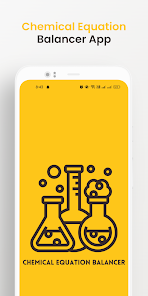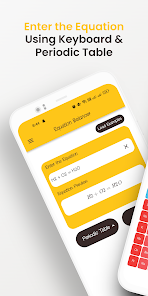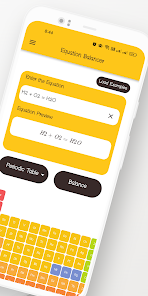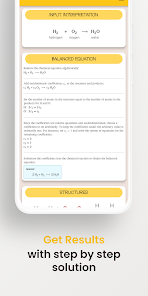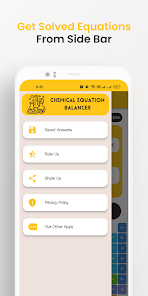An chemical equation solver is a software application that helps balance chemical reactions by adjusting the coefficients of reactants and products in a chemical equation. Chemical reactions are an integral part of chemistry and are used in various fields such as medicine, agriculture, and industry.

Balancing chemical equations is an important task in chemistry, but it can be time-consuming and prone to human error. Chemical Equation Balancing Calculator (chemistry calculator) provide a convenient and efficient solution to this problem.

How Does Chemical Equation Balancer App Work?

Following are the steps to Balance Chemical Equations using this app:

- An chemical equation solver uses algorithms to balance chemical reactions.

- To use the app of chem calculator, the user simply enters the unbalanced equation into the chemical balancer app, and the app adjusts the coefficients of the reactants and products to solve chemical equations.

- The balanced equation is then displayed as the result on Balancing chemicals calculator.

- The chemistry balancer app uses the mass balance principle, which states that the number of atoms of each element in a reaction must be equal on both sides of the equation.

Benefits of Using balancing chemical calculator

There are many benefits of using Chemistry Equation Balancing App, including:

Accuracy: Chemistry solver with chemical formulas eliminate the chance of human error and provide accurate balancing of chemical equations. This is especially important in chemistry, where even small mistakes can have significant consequences.

Speed: Balancing chemical equations can be time-consuming, but Balance chemical equation calculator can perform the task much faster, saving the user time and effort.

Convenience: Balance Chemistry Equations can be used anywhere, at any time, as long as the user has a device with them. This makes it easy for students, professionals, and anyone with an interest in chemistry to access the chemistry equation solver app.

Easy to Use: Most Equation Balancer (chem calculator) Apps have a user-friendly interface and are easy to use, even for those with limited knowledge of chemistry.

Saves Time: By eliminating the need for manual calculations, Chemical Balancer App can save a significant amount of time to measure chemistry formulas.

Improves Learning: Balancing chemicals calculator can be an excellent learning tool, helping students to understand chemical reactions and the balancing of chemical equations. No doubt, it is the best way to solve problems of equations and chemical reactions.

Increases Efficiency: chemistry balancer apps can make balancing chemical equations much more efficient, freeing up time for other important tasks.

Choosing Chemical Equation Balancer App

When choosing Chemical Equation Balancing Calculator, there are several factors to consider, including the app's accuracy, speed, user-friendly interface, and compatibility with your device. It is important to do research on how this app solve chemical equations and read reviews before making a decision to ensure that the balancing chemical calculator meets your needs.

Final Thoughts on Chemistry Equation Balancing App

A chemistry solver with chemical formulas is a convenient and efficient tool for balancing chemical reactions. By eliminating the chance of human error, saving time, and improving learning, Balance chemical equation calculator have become an essential tool for students, professionals, and anyone with an interest in chemistry for solving Chemistry Equations.

With so many options available on the market of chemistry calculators, it is important to choose an chemistry equation solver that meets your needs to Balance Chemical Equations and provides accurate and fast balancing of chemical equations.

So why are you still solving Chemistry Equations offline? Just download this chemical equation balancer app to solve problems of equations and chemical reactions.
Updated on
Apr 19, 2023

## Data safety

Safety starts with understanding how developers collect and share your data. Data privacy and security practices may vary based on your use, region, and age. The developer provided this information and may update it over time.No data shared with third parties